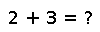# dawson

dawson(x)

Compute the Dawson function (scaled imaginary error function) of x, defined by $\frac{\sqrt{\pi}}{2} e^{-x^2} \operatorname{erfi}(x)$.

## Examples

julia> dawson(16)
0.031311396325184614


Checking you are not a robot: